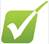Mass and moles questionsQu 1 - 3:  can you use the mole formula for masses                                   Qu 4 - 8:  Do you understand the concept of the mole

1)  What is the mass of (give your answer to 1dp) :­

a)                      0.1 moles of zirconium atoms Zr?

b)                      0.02 moles of Thorium atoms Th?

c)                      2.0 moles of Nickel atoms Ni?

d)                      0.1 moles of Mercury atoms Hg?

e)                      0.05 moles of Phosphorous molecules P4?

2) Calculate the mass of (give your answer to 1 dp):-

a)                      1.0 mole of H2?

b)                      1.0 mole of H?

c)                      1.0 mole of SiO2?

d)                      0.5 moles of NaCl?

e)                      0.25 moles of Na2CO3.10H2O?

3) How many moles of :­

a)                      32g of oxygen molecules?

b)                      32g of oxygen atoms?

c)                      31g of phosphorous molecules P4?

d)                      32.1g of sulphur molecules S8?

4) The formula of Sodiumdihydrido - bis ( 2 - methoxy ethoxy) Aluminate is abbreviated SDA for ease :­

NaAlH2(OCH2CH2OCH3)2

a)                      How many moles of Na atoms are there in 1 mole of SDA?

b)                      How many moles of carbon are there in 1 mole of SDA?

c)                      How many atoms of Al are there in 1 mole of SDA?

d)                      How many atoms of H are there in 1 mole of SDA?

e)                      What is the total number of moles of atoms of all the elements in 1moIe of SDA?

5) 1mole of sodium and 1 mole of iron must have the same:

Choose one:- a) mass     b) density     c) volume     d) number of atoms     e) number of electrons

6) Which of the following contains the greatest proportion of nitrogen by mass?

 a) Ammonium Sulphate (NH4)2SO4 Mr = 132 b) Ammonium carbonate (NH4)2CO3 Mr = 96 c) Ammonium nitrate NH4NO3 Mr = 80 d) Ammonium ethanoate CH3COONH4 Mr = 77 e) Ammonium chloride NH4Cl Mr = 53.5

7) The Avogodro number is 6.02 x 1023 which is the same number as:  ­Choose one

a)                      Hydrogen atoms in 18g of water.

b)                      Hydrogen molecules in 1g of Hydrogen.

c)                      Oxygen atoms in 44g of carbon dioxide.

d)                      Nitrogen molecules in 28g of nitrogen.

8) What mass of aluminium contains the same number of atoms as there are molecules of 22g of carbon dioxide?